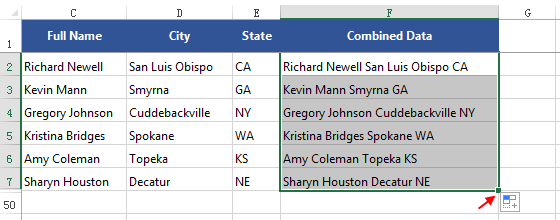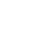» Resources » Office » How to Combine Cells Data into One in Excel

How to Combine Cells Data into One in Excel

The Concatenate function in Excel allows you to combine text from different cells into one cell. Here describes how to combine two or more cells data into one cell with formula.

To Combine text from two cells into one

In our example, we want to combine the text in cells A2 and B2 into C2.

Here we will enter following formula into cell C2:

=B2&A2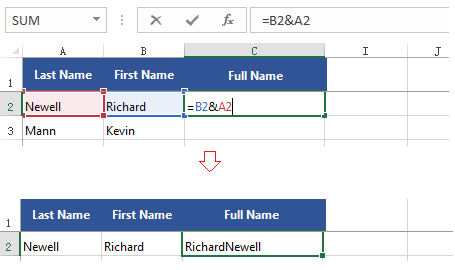To add spaces between each column, enter this formula:

=(A2&" "&B2)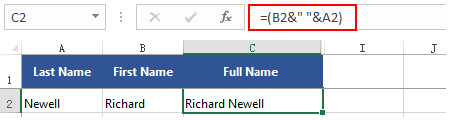To Combine multiple cells data into one cell

To combine data from cells more than two, such A2, B2 and C2.

=A1&B1&C1

To add spaces between each set of data, write this formula:

=(A2&" "&B2&" "&C2)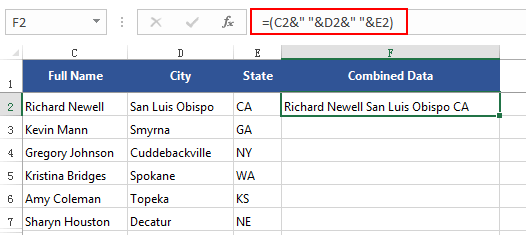To AutoFill in the rest cells use this formula

Drag the fill handle to copy the formula to the remaining cells in this column.